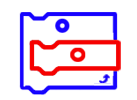# Coding Resources

Coding Number Mathematical Modelling Financial Literacy
All 1 2 3 4 5 6 7 8
Overall Lesson Webinar Recording Lesson Support
Select All    Clear All

NAME
CATEGORYGr 7/8 Webinar: Coding in the Intermediate Grades   [MP4]

Welcome to this webinar about teaching the new coding expectations for the intermediate grades of the revised mathematics curriculum. We will be taking you through the lessons that have been created as supports for Ontario educators as well as the pedagogy and social emotional learning skills that coding brings to your classroom.
NOTE: This recording does not yet contain the Q&A section - those will be added soon from all the recordings with captioning.

Webinar RecordingGr 4/5/6 Webinar: Coding in the Junior Grades   [MP4]

Welcome to this webinar about coding in the mathematics curriculum. We will be taking you through the pedagogy and social emotional learning skills that coding brings to your classroom as well as the lessons that have been created as supports for Ontario teachers in the Junior grades.
NOTE: This recording does not yet contain the Q&A section - those will be added soon from all the recordings with captioning.

Webinar RecordingGr 1/2/3 Webinar: Coding in the Primary Grades   [MP4]

Welcome to this webinar about coding in the mathematics curriculum. We will be taking you through the pedagogy and social emotional learning skills that coding brings to your classroom as well as the lessons that have been created as supports for Ontario teachers in the primary grades.
NOTE: This recording does not yet contain the Q&A section - those will be added soon from all the recordings with captioning.

Webinar Recording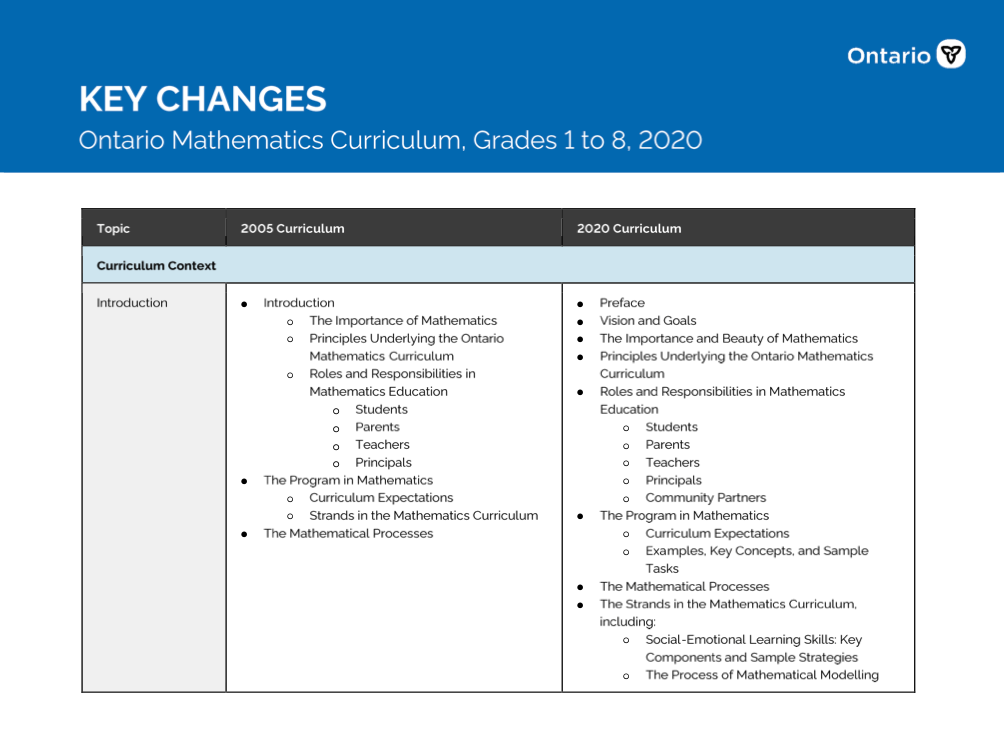Key Changes - Ontario Mathematics Curriculum, Grades 1 to 8, 2020   [PDF]

This document, prepared by the Ontario Ministry of Education, compares in table form the key changes between the 2005 and the 2020 elementary mathematics curriculum.

Overall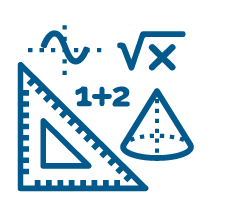Ontario Mathematics Curriculum, 2020, Grades 1 - 8   [URL]

This curriculum policy replaces The Ontario Curriculum, Grades 1-8: Mathematics, 2005. Beginning in September 2020, all mathematics programs for Grades 1 to 8 will be based on the expectations outlined in this curriculum policy.

OverallGrade 1 - Coding Lesson - Storybook Characters on a Grid   [Folder]

This is a series of 5 lessons, anchored in well known fairy tales, that invite students to learn pseudocoding through simple movements related to spatial reasoning.

LessonGrade 1 - Coding Lesson - Please direct me to my seat   [Folder]

In this lesson, students practice directing each other around different obstacles on a full-size grid to an end location by giving and receiving accurate direction.

LessonGrade 1 - Coding Lesson - Let's play hockey   [Folder]

In this lesson, students are challenged to find a route for a hockey player around numerous obstacles down the ice to the opposing team’s goal.

LessonGrade 1 - Coding Lesson - Barnyard Commotion and Motion   [Folder]

This is a series of three lessons, anchored in barnyard characters, that invite students to progress from using directional and positional language and physical movement to pseudocoding with numbers and arrows.

LessonGrade 1 - Coding Lesson - Hen Runs Away From Fox   [Folder]

Hen Runs Away From Fox: Children learn to animate a character (sprite) using Scratch or a similar block coding platform so that the character moves from left to right across a computer screen.

Lesson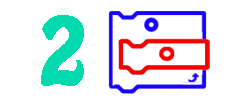Grade 2 - Coding Lesson - Coding with coins   [Folder]

In this lesson students will have to write unplugged codes using a sequence of actions on a 100's carpet or 10 x 10 grid to pick up enough coins that equal 75¢ to buy a marker.

LessonGrade 2 - Coding Lesson - Dancing to Code / Coding to Dance   [Folder]

In this lesson, the students will create and perform a set of dances in a chosen sequence containing precise unplugged instructions for performing the dances.

LessonGrade 2 - Coding Lesson - Building Number Sentences   [Folder]

In this lesson, students use coding devices to do plugged sequential coding to create number sentences while using a 100's chart.

LessonGrade 2 - Coding Lesson - Coding for the squirrel   [Folder]

In this lesson, students will read a story and help the squirrel to find, share and store acorns in the forest, and to problem solve using coding skills.

LessonGrade 3 - Coding Lesson - Location and Movement - Coding Ourselves   [Doc]

In this lesson students engage in an unplugged lesson that explores spatial sense (location and movement) as well as coding. There are also links to oral communications and the social studies map and globe skills continuum. Students will give their partner a set of directions that will have them move about the classroom using coding strategies.

LessonGrade 3 - Coding Lesson - Dance Patterns   [Folder]

In this lesson students will explore repeating patterns through dance using an online coding tool as well as with their own bodies. It explores coding skills as well as making cross curricular links to dance.

LessonGrade 3 - Coding Lesson - Making Change   [Folder]

In this lesson students will have opportunities to estimate change using coins as well as debug code. It explores financial literacy as well as coding.

LessonGrade 3 - Coding Lesson - Coding a Table of Values   [Folder]

In this lesson the students create tables of values representing growing and shrinking patterns to solve problems by remixing code. It addresses multiple expectations in the algebra strand.

Lesson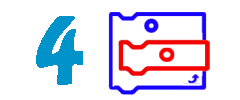Grade 4 - Coding Lesson - Coding the Way   [Folder]

Use coded directions to perform translations on a grid.

LessonGrade 4 - Coding Lesson - Dancing Our Way to Coding Using Patterning   [Doc]

Use physical movements and block coding to create repeating patterns to describe translations (sequential and loops).

LessonGrade 4 - Coding Lesson - Coding on a Coordinate Grid   [Folder]

Introduction to coding on a Cartesian plane using ordered pairs in the first quadrant; paper grid (unplugged coding) and Scratch

LessonGrade 4 - Coding Lesson - Let's Paint our Classroom   [Doc]

Students will create a coding formula in Scratch to find the area of a rectangle so they can paint the walls of the classroom. This lesson addresses multiple expectations in Spatial Sense and Financial Literacy.

LessonGrade 4 - Coding Lesson - Scratch your Probabilities!   [Doc]

Use mathematical vocabulary to make predictions of a probability (heads or tails) and use a code (Scratch) to represent this situation.

LessonGrade 5 - Coding Lesson - Budgets and Spreadsheets: Making plans   [Folder]

Coding using Financial Literacy - In this lesson, students will understand how spreadsheet programs can be used to manage a budget, alter a sample spreadsheet to meet our own plans, and learn to design budgets and make choices based on changing situations (for example by differentiating between needs and wants).

LessonGrade 5 - Coding Lesson - Navigational Coding - Unplugged   [Folder]

Coding through Location and Movement - In this lesson, students will create code to take a trip route using the coordinate plane, using shape, location and movement descriptors. They may even encounter detours along the way.

LessonGrade 5 - Coding Lesson - Recalling Math Facts through Coding   [Folder]

In this lesson, students will learn how to recall and demonstrate multiplication and division facts up to 12 x 12 by using and manipulating existing code. They will apply this code to a spreadsheet to create original artwork.

LessonGrade 5 - Coding Lesson - Exploring Patterns with fractions Using Scratch   [Doc]

In this lesson, using Scratch, students will learn to understand and represent patterns, relating them to equivalent decimals and fractions so they understand how the size of the whole affects the size of a fraction. They will also learn to make predictions about patterns.

LessonGrade 6 - Coding Lesson - Part 1: Getting Ready to Code Transformations - Unplugged!   [Folder]

In an unplugged environment, students will be learning to understand the movement of objects on the Cartesian plane through the graphing of ordered pairs (x, y) and using combinations of translations and reflections to transform objects. This lesson can be used in advance of Lesson 1, "Coding Transformations - Unplugged!"

LessonGrade 6 - Coding Lesson - Part 2: Coding Transformations - Unplugged   [Folder]

In an unplugged environment, students will be coding transformations--combinations of translations and reflections--on the Cartesian plane by writing and executing pseudocode.

LessonGrade 6 - Coding Lesson - Part 1: Which Integer is Greater?   [Folder]

Creating a pseudocode for the actions to determine which integer is greater than another, students then identify the Scratch coding blocks that will carry out these actions. They can remix a sample Scratch project or programme their own from a starter Scratch project. The use of a number line in the coding projects gives a visual representation to the integers. Note: For teachers not familiar with Scratch coding, instructions are included to assist your teaching of these lessons.

LessonGrade 6 - Coding Lesson - Part 2: Mystery Integers!   [Folder]

Creating a pseudocode for the actions to determine the actions used when using an "input/output machine" students then identify the Scratch coding blocks that will carry out these actions. They can remix a sample Scratch project or programme their own from a starter Scratch project. This lesson and Which is Greater? focus on developing your students' understanding of positive and negative integers. Note: For teachers not familiar with Scratch coding, instructions are included to assist your teaching of these lessons.

LessonGrade 6 - Coding Lesson - Start Small - Making Informed Financial Decisions   [Folder]

In this lesson, students will code using Google Sheets or any spreadsheet program. Students will be creating a budget to help plan for a financial goal while considering earnings and expenses.

LessonGrade 6 - Coding Lesson - Coding and Composite Numbers   [Folder]

Through the use of pseudocode, students will be learning to decompose their mathematical thinking regarding the concepts of prime and composite numbers and algorithms; identify the factors of composite numbers as a series of actions; connect those actions to block coding, and in doing so, reinforce their computational thinking. Note: Consider using coding lesson after the students understand the concept of factoring. For teachers not familiar with Scratch coding, instructions are included to assist your teaching of these lessons.

Lesson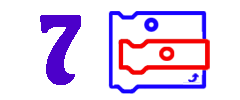Grade 7 - Coding Lesson - Get the Wolf to her Pups   [Folder]

In this unplugged coding lesson, students use computational thinking to move an object (the Wolf) in a cartesian plane using rotations and translations. The ultimate goal is to move the wolf so that she can join her pups. Student begin by writing instructions (code) to move the wolf freely without any obstacles, followed by adapting their code in order to avoid rivers and pits.

LessonGrade 7 - Coding Lesson - Restaurant Order app   [Folder]

A lesson in which students get to create a prototype for a restaurant ordering app. Using different conditional and operations blocs in Scratch, students get to work on expectations in the Numbers strand and calculate the total cost of a meal. Various scenarios (with different items and different quantities) allow for differentiation and various levels of scaffolding.

LessonGrade 7 - Coding Lesson - \$ 20 or 20%?   [Folder]

In this coding lesson using Scratch, students can create a calculator in which they can compare two different discount structures. Depending on the number of items bought, the calculator will be able to provide savings from either choosing a 20% discount, or a \$ 20 discount. Students get to utilise conditions and operations blocs in this activity.

LessonGrade 7 - Coding Lesson - Draw a maze   [Folder]

By the ways of math history and the beauty of math, students discover how math is useful in everyday life, including gaming! Students get to use Scratch to code a sequence to move an avatar through a maze. By gamifying the process, students are working on their understanding of translations, rotations, angles and distance. We are also asking them to think about the sequence of instructions (code) needed to move the avatar from point A to point B.

Lesson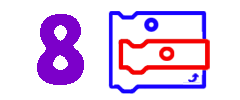Grade 8 - Coding Lesson - Transformations with Coding   [Folder]

In this lesson, students use their mapping rules to code transformations on a Cartesian Plane. Students will put their transformational geometry skills to work as they decipher existing code, find the bug and correct the error.

LessonGrade 8 - Coding Lesson - Coding and Geometry   [Folder]

Become a Scratch artist! In this lesson, students navigate through the block coding platform to perform reflections, translation and rotations on a variety of polygons. Students will learn to not only read and interpret lines of code but will learn to create their own.

LessonGrade 8 - Coding Lesson - Coding with the Pythagorean Theorem   [Doc]

In this lesson, students will increase their knowledge of the Pythagorean Theorem while working on their coding skills.

LessonGrade 8 - Coding Lesson - Coding YouTube   [Doc]

Do your students want to become YouTube stars? Students will learn how to Monetize a YouTube Channel and use coding to predict the revenue a YouTube video can generate.

Lesson

Lesson - Infinity Pool - Using Geometry and Coding to Explore Limits and Infinity   [GDoc]

This lesson introduces students to the concepts of limits and infinity through the lens of a practical geometry and measurement problem and through the use of coding and spreadsheets. Students will begin with a practical problem, then investigate, in a theoretical way, how increasing the number of sides of a regular polygon, while holding the perimeter fixed, influences the area of the polygon. They will create a pattern of polygon area calculations that tends to the largest area, a circle, by adding smaller and smaller increments. Patterns will be examined using tables of values and graphs, with an end goal of students understanding that the area will approach a limiting value. Students will then apply this new knowledge to the original practical problem.

Lesson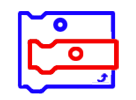Coding Glossary   [PDF]

A bilingual compilation of pertinent vocabulary directly related to computational thinking and computer programming/coding.

Lesson SupportInfographic - Coding and the 2020 Math Curriculum   [PDF]

A continuum from Grades 1-8 outlining the key coding concepts per grade coupled with a Scratch project with teacher notes.

Lesson Support